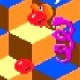#Note: Translated words like ‘Oscar Fingal O’Flahertie Wills Wilde’ indicate unusual relationships between the character positions in the word(s) being translated and result of the translation.

God (UFO/ET) talks to us through the ET Corn Gods language translation of words.

Oscar Fingal O’Flahertie Wills Wilde:
Oscar:
Os c ar = “Raso”.
V is 22, 2+2 = 4, d.
Rev is 1854.
Therefore:
R = “1854”
Therefore:
D is 500, 50, L, 12.
1 is one, On Date. (e is 5, book dt, t is bo, b+o, 17. 7+66+66 … = “te”)
B is Boron, “Born”. (Drop “o”)
A is 1, 100, C, 30, 16+10, 16 October.
Therefore:
Oscar = “1854 AD, on date born, 16 Ocober So”.
So is Os, backwards, “Osmium:
Backwards is “Osmim”.
O is 15, 15+66 = “Ha”, H+A = “I”.
Add 0+66, book rev, v is 5, 50, L.
Therefore:
Osmim = “Iremim”.
M is l+a, “La”.
Therefore:
Oscar = “1854 AD, on date born, 16 October Irishman”.
Fingal:

Add 0+66+66 … = “or”, backwards is Ro, book 45, backwards is 54, 54+66 = “120”, “At”
6 = 46.
I is 4+5.
Therefore:
Al:
L+66+66 = “nd”, N+d = r, r+66+66… = “al”, backwards is La, 121, backwards is 121, “La”, book 25.
Therefore;
Fingal = “At 46 Dead, Gay”.
Add 0+66+66 .. = “ro”, backwards is or, book 45, 45+66 = “111”, “Ck”, “30 November”.
O’Flahertie:
O+66 = “ha”.
Therefore:
of = “Wahef”, “wref”
Ef+66+66 = “in”.
Therefore:
Of = “Wri n”
Add 0+66+66 .. = “Sn”, “Tin”, “Ter”. (in-66-66 .. = “Er”)
Therefore:
O’Flahertie = “Writer nflahertie”.
Nf is AD 6, book Ro, “R”. (drop “o”)
R is 18, Job. Ob is book 31, “P+O”,
La is 121, backwards is 121, La, book 25, backwards is 52, 520, “ET”.
Therefore:
O’Flahertie = “Writer 10 Poet hertie”.
Hertie:
R is 2+16.
Heb is he-66, S.
B is 2, book of Ex.
Therefore:
Hertie = “Sex ptie”.
Netie:
Net cancels with Ten.
I is 9, ix, i1212, i5050, ic.
Ice = “ei”, 59, book James = “Games”. (J is Jo, backwards is oj, oj-66-66 …. = “ET”, backwards is Te, te-66-66 .. = “G”)
Therefore:
O’Flahertie = “Writer Poet Sex Games”.
O’Flahertie:
O is 15, book “Ezr”.
Z is 26, 2+6, 8.
58 is book Heb.
He-66 = “S”.
B is 2, book “ex”
R is 18, book “Job”.
Therefore:
0 = “Sex Job”.
O’Flahertie = “Sex Job Flam rtie”.
R is Ro, book 45, 20+25, “Boy”.
Therefore:
O’Flahertie = “Sex Job, Flamboy tie”.
Therefore;
O’Flahertie = “Sex Job, Flamboyant ie”.
Ie = d+5ee
D is “Dress”. (add 0+66, book Rev, v is 22, book ss)
Therefore:
Flahertie = “Flamboyant Dress eee”.
E+66 = “Ga”.
Ae is o, drop it.
E is 5, 55, le, abe, ay. (five = I-f ve = cve, ee).
Therefore:
O’Fahertie = “Sex Job, Flamboyant Dress Gay”.
Wills Wilde:
W is 23, 5, 500, “D”.
Therefore:
Wills = “Dorian lls”.
L is 50, 500, D, d+66 = “70”, “7”, “G”.
L+66+66 = “nd”, n+d = “R”.
S is 19, backwards is 91, 91-66 = “be”, book La, 121, backward is 121.
121-66 = “55”, Le, “125”, “ay”.
Therefore:
Wills = “Dorian Gray”
E:
Re-66 = “as”.
Add 0+66+66 .. = “33”, “CC”
Ac is m.
Add 0+66+66 . = “924”, Ix, nine, “I”.
Cs is 119, “as”.
E is e+66+66 .. = “Movie”.
Wills Wilde = “Dorian Gray Wild Movie”.

## Oscar Fingal O’Flahertie Wills Wilde = “1854 AD, on date born, 16 October Irishman, At 46 November 30, 1900 AD, Dead, Gay, Writer Poet Sex Games, Sex Job, Flamboyant Dress Gay, Dorian Gray Wild Movie”

How does George R. Simpson do these translations? See [HERE]

## Visit:

www.ETCornGods.com

## 4 thoughts on “The words “Oscar Fingal O’Flahertie Wills Wilde”.”

1. Pingback: The word “Event”. | UfoEtBlog.com

2.Squazz Muntbacker on said:

1985?

•admin on said:

Thanks, fixed it to read “1854”.